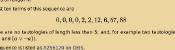mscroggs.co.uk
mscroggs.co.uksubscribe

# Blog

## How OEISbot works

2015-08-29
A few weeks ago, I made OEISbot, a Reddit bot which posts information whenever an OEIS sequence is mentioned.
This post explains how OEISbot works. The full code can be found on GitHub.

### Getting started

OEISbot is made in Python using PRAW (Python Reddit Api Wrapper). PRAW can be installed with:
bash
pip install praw
Before making a bot, you will need to make a Reddit account for your bot, create a Reddit app and obtain API keys. This python script can be used to obtain the necessary keys.
Once you have your API keys saved in your praw.ini file, you are ready to make a bot.

### Writing the bot

First, the necessary imports are made, and test mode is activated if the script is run with test as an argument. We also define an exception that will be used later to kill the script once it makes a comment.
python
import praw
import re
import urllib
import json
from praw.objects import MoreComments

import sys
test = False
if len(sys.argv) > 1 and sys.argv == "test":
test = True
print("TEST MODE")

class FoundOne(BaseException):
pass

To prevent OEISbot from posting multiple links to the same sequence in a thread, lists of sequences linked to in each thread can be loaded and saved using the following functions.
python
def save_list(seen, _id):
print(seen)
with open("/home/pi/OEISbot/seen/"+_id, "w"as f:
return json.dump(seen, f)

def open_list(_id):
try:
with open("/home/pi/OEISbot/seen/" + _id) as f:
return json.load(f)
except:
return []
The following function will search a post for a mention of an OEIS sequence number.
python
def look_for_A(id_, text, url, comment):
seen = open_list(id_)
re_s = re.findall("A([0-9]{6})", text)
re_s += re.findall("oeis\.org/A([0-9]{6})", url)
if test:
print(re_s)
post_me = []
for seq_n in re_s:
if seq_n not in seen:
post_me.append(markup(seq_n))
seen.append(seq_n)
if len(post_me) > 0:
post_me.append(me())
comment(joiner().join(post_me))
save_list(seen, id_)
raise FoundOne
The following function will search a post for a comma-separated list of numbers, then search for it on the OEIS. If there are 14 sequences or less found, it will reply. If it finds a list with no matches on the OEIS, it will message /u/PeteOK, as he likes hearing about possibly new sequences.
python
def look_for_ls(id_, text, comment, link, message):
seen = open_list(id_)
if test:
print(text)
re_s = re.findall("([0-9]+\, *(?:[0-9]+\, *)+[0-9]+)", text)
if len(re_s) > 0:
for terms in ["".join(i.split(" ")) for i in re_s]:
if test:
print(terms)
if terms not in seen:
seen.append(terms)
first10, total = load_search(terms)
if test:
print(first10)
if len(first10)>and total <= 14:
if total == 1:
intro = "Your sequence (" + terms \
+ ") looks like the following OEIS sequence."
else:
intro = "Your sequence (" + terms + \
+ ") may be one of the following OEIS sequences."
if total > 4:
intro += " Or, it may be one of the " + str(total-4) \
+ " other sequences listed [here]" \
"(http://oeis.org/search?q=" + terms + ")."
post_me = [intro]
if test:
print(first10)
for seq_n in first10[:4]:
post_me.append(markup(seq_n))
seen.append(seq_n)
post_me.append(me())
comment(joiner().join(post_me))
save_list(seen, id_)
raise FoundOne
elif len(first10) == 0:
post_me = ["I couldn't find your sequence (" + terms \
+ ") in the [OEIS](http://oeis.org). "
"You should add it!"]
message("PeteOK",
"Sequence not in OEIS",
"Hi Peter, I've just found a new sequence (" \
+ terms + ") in [this thread](link). " \
"Please shout at /u/mscroggs to turn the " \
"feature off if its spamming you!")
post_me.append(me())
comment(joiner().join(post_me))
save_list(seen, id_)
raise FoundOne

def load_search(terms):
src = urllib.urlopen("http://oeis.org/search?fmt=data&q="+terms).read()
ls = re.findall("href=(?:'|\")/A([0-9]{6})(?:'|\")", src)
try:
tot = int(re.findall("of ([0-9]+) results found", src))
except:
tot = 0
return ls, tot
The markup function loads the necessary information from OEIS and formats it. Each comment will end with the output of the me function. The ouput of joiner will be used between sequences which are mentioned.
python
def markup(seq_n):
pattern = re.compile("%N (.*?)<", re.DOTALL|re.M)
desc = urllib.urlopen("http://oeis.org/A" + seq_n + "/internal").read()
desc = pattern.findall(desc).strip("\n")
pattern = re.compile("%S (.*?)<", re.DOTALL|re.M)
seq = urllib.urlopen("http://oeis.org/A" + seq_n + "/internal").read()
seq = pattern.findall(seq).strip("\n")
new_com = "[A" + seq_n + "](http://oeis.org/A" + seq_n + "/): "
new_com += desc + "\n\n"
new_com += seq + "..."
return new_com

def me():
return "I am OEISbot. I was programmed by /u/mscroggs. " \
"[How I work](http://mscroggs.co.uk/blog/20). " \
"You can test me and suggest new features at /r/TestingOEISbot/."

def joiner():
return "\n\n- - - -\n\n"
Next, OEISbot logs into Reddit.
python
= praw.Reddit("OEIS link and description poster by /u/mscroggs.")

access_i = r.refresh_access_information(refresh_token=r.refresh_token)
r.set_access_credentials(**access_i)

auth = r.get_me()
The subs which OEISbot will search through are listed. I have used all the math(s) subs which I know about, as these will be the ones mentioning sequences.
python
subs = ["TestingOEISbot","math","mathpuzzles","casualmath","theydidthemath",
"learnmath","mathbooks","cheatatmathhomework","matheducation",
"puremathematics","mathpics","mathriddles","askmath",
"recreationalmath","OEIS","mathclubs","maths"]
if test:
subs = ["TestingOEISbot"]
For each sub OEISbot is monitoring, the hottest 10 posts are searched through for mentions of sequences. If a mention is found, a reply is generated and posted, then the FoundOne exception will be raised to end the code.
python
try:
for sub in subs:
print(sub)
subreddit = r.get_subreddit(sub)
for submission in subreddit.get_hot(limit = 10):
if test:
print(submission.title)
look_for_A(submission.id,
submission.title + "|" + submission.selftext,
submission.url,
submission.add_comment)
look_for_ls(submission.id,
submission.title + "|" + submission.selftext,
submission.add_comment,
submission.url,
r.send_message)

flat_comments = praw.helpers.flatten_tree(submission.comments)
for comment in flat_comments:
if ( not isinstance(comment, MoreComments)
and comment.author is not None
and comment.author.name != "OEISbot" ):
look_for_A(submission.id,
re.sub("$[^$]*\]$$[^$$*]\)","",comment.body),
comment.body,
comment.reply)
look_for_ls(submission.id,
re.sub("$[^$]*\]$$[^$$*]\)","",comment.body),
comment.reply,
submission.url,
r.send_message)

except FoundOne:
pass

### Running the code

I put this script on a Raspberry Pi which runs it every 10 minutes (to prevent OEISbot from getting refusals for posting too often). This is achieved with a cron job.
bash
*/10 * * * * python /path/to/bot.py

### Making your own bot

The full OEISbot code is available on GitHub. Feel free to use it as a starting point to make your own bot! If your bot is successful, let me know about it in the comments below or on Twitter.
Edit: Updated to describe the latest version of OEISbot.

### Similar postsLogic botRaspberry Pi weather stationLogical contradictionsLogic bot, pt. 2

### Comments

Comments in green were written by me. Comments in blue were not written by me.
Add a Comment

I will only use your email address to reply to your comment (if a reply is needed).

Allowed HTML tags: <br> <a> <small> <b> <i> <s> <sup> <sub> <u> <spoiler> <ul> <ol> <li>
To prove you are not a spam bot, please type "s" then "e" then "g" then "m" then "e" then "n" then "t" in the box below (case sensitive):

## Archive

Show me a random blog post
▼ show ▼
© Matthew Scroggs 2012–2021